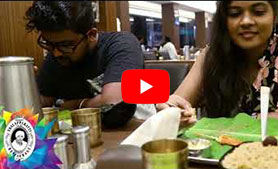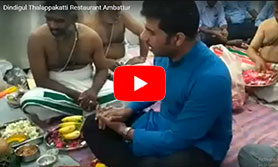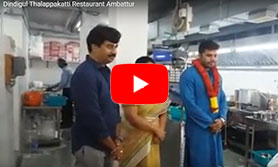## MEDIA COVERAGE

#### Videos

```/* <![CDATA[ */
\$(function(){
var width = \$(window).innerWidth();
var setwidth = 640;
var ratio = 0.75;
var height = 480;
if(width < setwidth)
{
height = Math.floor(width * 0.75);
//console.log("device width "+width+", set width "+640+", ratio "+0.75+", new height "+ height);
var new_url = wpvl_paramReplace('width', link, width);
var new_url = wpvl_paramReplace('height', new_url, height);
\$("a#5fc93eb8965db").attr('href', new_url);
//console.log(new_url);
}
});
});
/* ]]&gt; */
```

Nagercoil Branch

```/* <![CDATA[ */
\$(function(){
var width = \$(window).innerWidth();
var setwidth = 640;
var ratio = 0.75;
var height = 480;
if(width < setwidth)
{
height = Math.floor(width * 0.75);
//console.log("device width "+width+", set width "+640+", ratio "+0.75+", new height "+ height);
var new_url = wpvl_paramReplace('width', link, width);
var new_url = wpvl_paramReplace('height', new_url, height);
\$("a#5fc93eb896a25").attr('href', new_url);
//console.log(new_url);
}
});
});
/* ]]&gt; */
```

Ulundurpet Branch

```/* <![CDATA[ */
\$(function(){
var width = \$(window).innerWidth();
var setwidth = 640;
var ratio = 0.75;
var height = 480;
if(width < setwidth)
{
height = Math.floor(width * 0.75);
//console.log("device width "+width+", set width "+640+", ratio "+0.75+", new height "+ height);
var new_url = wpvl_paramReplace('width', link, width);
var new_url = wpvl_paramReplace('height', new_url, height);
\$("a#5fc93eb896ec9").attr('href', new_url);
//console.log(new_url);
}
});
});
/* ]]&gt; */
```

Perambur Branch

```/* <![CDATA[ */
\$(function(){
var width = \$(window).innerWidth();
var setwidth = 640;
var ratio = 0.75;
var height = 480;
if(width < setwidth)
{
height = Math.floor(width * 0.75);
//console.log("device width "+width+", set width "+640+", ratio "+0.75+", new height "+ height);
var new_url = wpvl_paramReplace('width', link, width);
var new_url = wpvl_paramReplace('height', new_url, height);
\$("a#5fc93eb8972c2").attr('href', new_url);
//console.log(new_url);
}
});
});
/* ]]&gt; */
```

Ambattur Branch Auspicious Puja Part 1

```/* <![CDATA[ */
\$(function(){
var width = \$(window).innerWidth();
var setwidth = 640;
var ratio = 0.75;
var height = 480;
if(width < setwidth)
{
height = Math.floor(width * 0.75);
//console.log("device width "+width+", set width "+640+", ratio "+0.75+", new height "+ height);
var new_url = wpvl_paramReplace('width', link, width);
var new_url = wpvl_paramReplace('height', new_url, height);
\$("a#5fc93eb897c2d").attr('href', new_url);
//console.log(new_url);
}
});
});
/* ]]&gt; */
```

Ambattur Branch Auspicious Puja Part 2

```/* <![CDATA[ */
\$(function(){
var width = \$(window).innerWidth();
var setwidth = 640;
var ratio = 0.75;
var height = 480;
if(width < setwidth)
{
height = Math.floor(width * 0.75);
//console.log("device width "+width+", set width "+640+", ratio "+0.75+", new height "+ height);
var new_url = wpvl_paramReplace('width', link, width);
var new_url = wpvl_paramReplace('height', new_url, height);
\$("a#5fc93eb898067").attr('href', new_url);
//console.log(new_url);
}
});
});
/* ]]&gt; */
```

Sun News Thinnai Program

```/* <![CDATA[ */
\$(function(){
var width = \$(window).innerWidth();
var setwidth = 640;
var ratio = 0.75;
var height = 480;
if(width < setwidth)
{
height = Math.floor(width * 0.75);
//console.log("device width "+width+", set width "+640+", ratio "+0.75+", new height "+ height);
var new_url = wpvl_paramReplace('width', link, width);
var new_url = wpvl_paramReplace('height', new_url, height);
\$("a#5fc93eb898477").attr('href', new_url);
//console.log(new_url);
}
});
});
/* ]]&gt; */
```

Food Distribution at Sirumalai

```/* <![CDATA[ */
\$(function(){
var width = \$(window).innerWidth();
var setwidth = 640;
var ratio = 0.75;
var height = 480;
if(width < setwidth)
{
height = Math.floor(width * 0.75);
//console.log("device width "+width+", set width "+640+", ratio "+0.75+", new height "+ height);
var new_url = wpvl_paramReplace('width', link, width);
var new_url = wpvl_paramReplace('height', new_url, height);
\$("a#5fc93eb89888d").attr('href', new_url);
//console.log(new_url);
}
});
});
/* ]]&gt; */
```

```/* <![CDATA[ */
\$(function(){
var width = \$(window).innerWidth();
var setwidth = 640;
var ratio = 0.75;
var height = 480;
if(width < setwidth)
{
height = Math.floor(width * 0.75);
//console.log("device width "+width+", set width "+640+", ratio "+0.75+", new height "+ height);
var new_url = wpvl_paramReplace('width', link, width);
var new_url = wpvl_paramReplace('height', new_url, height);
\$("a#5fc93eb899217").attr('href', new_url);
//console.log(new_url);
}
});
});
/* ]]&gt; */
```

Oasis Hostel Day Function in Madurai

```/* <![CDATA[ */
\$(function(){
var width = \$(window).innerWidth();
var setwidth = 640;
var ratio = 0.75;
var height = 480;
if(width < setwidth)
{
height = Math.floor(width * 0.75);
//console.log("device width "+width+", set width "+640+", ratio "+0.75+", new height "+ height);
var new_url = wpvl_paramReplace('width', link, width);
var new_url = wpvl_paramReplace('height', new_url, height);
\$("a#5fc93eb8995e4").attr('href', new_url);
//console.log(new_url);
}
});
});
/* ]]&gt; */
```

Indian Street Food Tour in Chennai

```/* <![CDATA[ */
\$(function(){
var width = \$(window).innerWidth();
var setwidth = 640;
var ratio = 0.75;
var height = 480;
if(width < setwidth)
{
height = Math.floor(width * 0.75);
//console.log("device width "+width+", set width "+640+", ratio "+0.75+", new height "+ height);
var new_url = wpvl_paramReplace('width', link, width);
var new_url = wpvl_paramReplace('height', new_url, height);
\$("a#5fc93eb8999e3").attr('href', new_url);
//console.log(new_url);
}
});
});
/* ]]&gt; */
```

How To Eat A Biriyani

```/* <![CDATA[ */
\$(function(){
var width = \$(window).innerWidth();
var setwidth = 640;
var ratio = 0.75;
var height = 480;
if(width < setwidth)
{
height = Math.floor(width * 0.75);
//console.log("device width "+width+", set width "+640+", ratio "+0.75+", new height "+ height);
var new_url = wpvl_paramReplace('width', link, width);
var new_url = wpvl_paramReplace('height', new_url, height);
\$("a#5fc93eb899df9").attr('href', new_url);
//console.log(new_url);
}
});
});
/* ]]&gt; */
```

Dindigul Thalappakatti Coverage in Podhigai

```/* <![CDATA[ */
\$(function(){
var width = \$(window).innerWidth();
var setwidth = 640;
var ratio = 0.75;
var height = 480;
if(width < setwidth)
{
height = Math.floor(width * 0.75);
//console.log("device width "+width+", set width "+640+", ratio "+0.75+", new height "+ height);
var new_url = wpvl_paramReplace('width', link, width);
var new_url = wpvl_paramReplace('height', new_url, height);
\$("a#5fc93eb89a7d3").attr('href', new_url);
//console.log(new_url);
}
});
});
/* ]]&gt; */
```

Revealing the secret of Dindigul Thalappakatti

```/* <![CDATA[ */
\$(function(){
var width = \$(window).innerWidth();
var setwidth = 640;
var ratio = 0.75;
var height = 480;
if(width < setwidth)
{
height = Math.floor(width * 0.75);
//console.log("device width "+width+", set width "+640+", ratio "+0.75+", new height "+ height);
var new_url = wpvl_paramReplace('width', link, width);
var new_url = wpvl_paramReplace('height', new_url, height);
\$("a#5fc93eb89ac72").attr('href', new_url);
//console.log(new_url);
}
});
});
/* ]]&gt; */
```

Making of Dindigul Thalappakatti – The Legend

```/* <![CDATA[ */
\$(function(){
var width = \$(window).innerWidth();
var setwidth = 640;
var ratio = 0.75;
var height = 480;
if(width < setwidth)
{
height = Math.floor(width * 0.75);
//console.log("device width "+width+", set width "+640+", ratio "+0.75+", new height "+ height);
var new_url = wpvl_paramReplace('width', link, width);
var new_url = wpvl_paramReplace('height', new_url, height);
\$("a#5fc93eb89b08e").attr('href', new_url);
//console.log(new_url);
}
});
});
/* ]]&gt; */
```

Dindigul Thalappakatti Restaurant TVC ft. Legendary Founder in 3D Animated Avatar

```/* <![CDATA[ */
\$(function(){
var width = \$(window).innerWidth();
var setwidth = 640;
var ratio = 0.75;
var height = 480;
if(width < setwidth)
{
height = Math.floor(width * 0.75);
//console.log("device width "+width+", set width "+640+", ratio "+0.75+", new height "+ height);
var new_url = wpvl_paramReplace('width', link, width);
var new_url = wpvl_paramReplace('height', new_url, height);
\$("a#5fc93eb89b4cc").attr('href', new_url);
//console.log(new_url);
}
});
});
/* ]]&gt; */
```

Dindigul Thalappakatti – The Legend

```/* <![CDATA[ */
\$(function(){
var width = \$(window).innerWidth();
var setwidth = 640;
var ratio = 0.75;
var height = 480;
if(width < setwidth)
{
height = Math.floor(width * 0.75);
//console.log("device width "+width+", set width "+640+", ratio "+0.75+", new height "+ height);
var new_url = wpvl_paramReplace('width', link, width);
var new_url = wpvl_paramReplace('height', new_url, height);
\$("a#5fc93eb89bdf2").attr('href', new_url);
//console.log(new_url);
}
});
});
/* ]]&gt; */
```

Opening of 21st Outlet @ Dinigul

```/* <![CDATA[ */
\$(function(){
var width = \$(window).innerWidth();
var setwidth = 640;
var ratio = 0.75;
var height = 480;
if(width < setwidth)
{
height = Math.floor(width * 0.75);
//console.log("device width "+width+", set width "+640+", ratio "+0.75+", new height "+ height);
var new_url = wpvl_paramReplace('width', link, width);
var new_url = wpvl_paramReplace('height', new_url, height);
\$("a#5fc93eb89c236").attr('href', new_url);
//console.log(new_url);
}
});
});
/* ]]&gt; */
```

What India EATS food Vlog

```/* <![CDATA[ */
\$(function(){
var width = \$(window).innerWidth();
var setwidth = 640;
var ratio = 0.75;
var height = 480;
if(width < setwidth)
{
height = Math.floor(width * 0.75);
//console.log("device width "+width+", set width "+640+", ratio "+0.75+", new height "+ height);
var new_url = wpvl_paramReplace('width', link, width);
var new_url = wpvl_paramReplace('height', new_url, height);
\$("a#5fc93eb89c633").attr('href', new_url);
//console.log(new_url);
}
});
});
/* ]]&gt; */
```

Opening of 21st Outlet @ Dindigul

```/* <![CDATA[ */
\$(function(){
var width = \$(window).innerWidth();
var setwidth = 640;
var ratio = 0.75;
var height = 480;
if(width < setwidth)
{
height = Math.floor(width * 0.75);
//console.log("device width "+width+", set width "+640+", ratio "+0.75+", new height "+ height);
var new_url = wpvl_paramReplace('width', link, width);
var new_url = wpvl_paramReplace('height', new_url, height);
\$("a#5fc93eb89ca6c").attr('href', new_url);
//console.log(new_url);
}
});
});
/* ]]&gt; */
```

Chennai International Fashion Week @ Thalappakatti

```/* <![CDATA[ */
\$(function(){
var width = \$(window).innerWidth();
var setwidth = 640;
var ratio = 0.75;
var height = 480;
if(width < setwidth)
{
height = Math.floor(width * 0.75);
//console.log("device width "+width+", set width "+640+", ratio "+0.75+", new height "+ height);
var new_url = wpvl_paramReplace('width', link, width);
var new_url = wpvl_paramReplace('height', new_url, height);
\$("a#5fc93eb89d416").attr('href', new_url);
//console.log(new_url);
}
});
});
/* ]]&gt; */
```

Dindigul Thalappakatti TV Commercial

```/* <![CDATA[ */
\$(function(){
var width = \$(window).innerWidth();
var setwidth = 640;
var ratio = 0.75;
var height = 480;
if(width < setwidth)
{
height = Math.floor(width * 0.75);
//console.log("device width "+width+", set width "+640+", ratio "+0.75+", new height "+ height);
var new_url = wpvl_paramReplace('width', link, width);
var new_url = wpvl_paramReplace('height', new_url, height);
\$("a#5fc93eb89d858").attr('href', new_url);
//console.log(new_url);
}
});
});
/* ]]&gt; */
```

Makkal TV – Rasikka Rusikka

```/* <![CDATA[ */
\$(function(){
var width = \$(window).innerWidth();
var setwidth = 640;
var ratio = 0.75;
var height = 480;
if(width < setwidth)
{
height = Math.floor(width * 0.75);
//console.log("device width "+width+", set width "+640+", ratio "+0.75+", new height "+ height);
var new_url = wpvl_paramReplace('width', link, width);
var new_url = wpvl_paramReplace('height', new_url, height);
\$("a#5fc93eb89dd18").attr('href', new_url);
//console.log(new_url);
}
});
});
/* ]]&gt; */
```

Dindigul Thalappakatti Coverage in Sathiyam TV – Win I Thoduvom (18.12.2013)

```/* <![CDATA[ */
\$(function(){
var width = \$(window).innerWidth();
var setwidth = 640;
var ratio = 0.75;
var height = 480;
if(width < setwidth)
{
height = Math.floor(width * 0.75);
//console.log("device width "+width+", set width "+640+", ratio "+0.75+", new height "+ height);
var new_url = wpvl_paramReplace('width', link, width);
var new_url = wpvl_paramReplace('height', new_url, height);
\$("a#5fc93eb89e1ae").attr('href', new_url);
//console.log(new_url);
}
});
});
/* ]]&gt; */
```

Dindigul Thalappakatti Coverage in Sathiyam TV – Win I Thoduvom (19.12.2013)

```/* <![CDATA[ */
\$(function(){
var width = \$(window).innerWidth();
var setwidth = 640;
var ratio = 0.75;
var height = 480;
if(width < setwidth)
{
height = Math.floor(width * 0.75);
//console.log("device width "+width+", set width "+640+", ratio "+0.75+", new height "+ height);
var new_url = wpvl_paramReplace('width', link, width);
var new_url = wpvl_paramReplace('height', new_url, height);
\$("a#5fc93eb89ebc8").attr('href', new_url);
//console.log(new_url);
}
});
});
/* ]]&gt; */
```

Dindigul Thalppakatti Coverage in Sun News

```/* <![CDATA[ */
\$(function(){
var width = \$(window).innerWidth();
var setwidth = 640;
var ratio = 0.75;
var height = 480;
if(width < setwidth)
{
height = Math.floor(width * 0.75);
//console.log("device width "+width+", set width "+640+", ratio "+0.75+", new height "+ height);
var new_url = wpvl_paramReplace('width', link, width);
var new_url = wpvl_paramReplace('height', new_url, height);
\$("a#5fc93eb89f029").attr('href', new_url);
//console.log(new_url);
}
});
});
/* ]]&gt; */
```

Dindigul Thalappakatti Coverage in Raj Tv

```/* <![CDATA[ */
\$(function(){
var width = \$(window).innerWidth();
var setwidth = 640;
var ratio = 0.75;
var height = 480;
if(width < setwidth)
{
height = Math.floor(width * 0.75);
//console.log("device width "+width+", set width "+640+", ratio "+0.75+", new height "+ height);
var new_url = wpvl_paramReplace('width', link, width);
var new_url = wpvl_paramReplace('height', new_url, height);
\$("a#5fc93eb89f3f3").attr('href', new_url);
//console.log(new_url);
}
});
});
/* ]]&gt; */
```

Thalappakatti featured in Food Food

```/* <![CDATA[ */
\$(function(){
var width = \$(window).innerWidth();
var setwidth = 640;
var ratio = 0.75;
var height = 480;
if(width < setwidth)
{
height = Math.floor(width * 0.75);
//console.log("device width "+width+", set width "+640+", ratio "+0.75+", new height "+ height);
var new_url = wpvl_paramReplace('width', link, width);
var new_url = wpvl_paramReplace('height', new_url, height);
\$("a#5fc93eb89f843").attr('href', new_url);
//console.log(new_url);
}
});
});
/* ]]&gt; */
```

Dindigul Thalappakatti Coverage in Puthiya Thalamurai

```/* <![CDATA[ */
\$(function(){
var width = \$(window).innerWidth();
var setwidth = 640;
var ratio = 0.75;
var height = 480;
if(width < setwidth)
{
height = Math.floor(width * 0.75);
//console.log("device width "+width+", set width "+640+", ratio "+0.75+", new height "+ height);
var new_url = wpvl_paramReplace('width', link, width);
var new_url = wpvl_paramReplace('height', new_url, height);
\$("a#5fc93eb8a01a1").attr('href', new_url);
//console.log(new_url);
}
});
});
/* ]]&gt; */
```

Dindigul Thalappakatti Biriyani Coverage in NDTV

```/* <![CDATA[ */
\$(function(){
var width = \$(window).innerWidth();
var setwidth = 640;
var ratio = 0.75;
var height = 480;
if(width < setwidth)
{
height = Math.floor(width * 0.75);
//console.log("device width "+width+", set width "+640+", ratio "+0.75+", new height "+ height);
var new_url = wpvl_paramReplace('width', link, width);
var new_url = wpvl_paramReplace('height', new_url, height);
\$("a#5fc93eb8a05d5").attr('href', new_url);
//console.log(new_url);
}
});
});
/* ]]&gt; */
```

Dindigul Thalappakatti Coverage in Sun Music

#### Blog

##### For online orders:Scan here